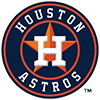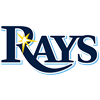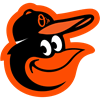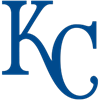DraftKings AL Championship Odds
+175
1.75 to 1Yankees
30.1% implied probability

No changes have been recorded yet.
+450
4.5 to 1Astros
15.1% implied probability

+650
6.5 to 1Twins
11.0% implied probability

+750
7.5 to 1Rays
9.7% implied probability

+1000
10 to 1Athletics
7.5% implied probability

+1200
12 to 1Indians
6.4% implied probability

+1400
14 to 1White Sox
5.5% implied probability

+1700
17 to 1Angels
4.6% implied probability

+2000
20 to 1Red Sox
3.9% implied probability

+3900
39 to 1Rangers
2.1% implied probability

+4400
44 to 1Blue Jays
1.8% implied probability

+12000
120 to 1Mariners
0.7% implied probability

+17000
170 to 1Orioles
0.5% implied probability

+17000
170 to 1Tigers
0.5% implied probability

+17000
170 to 1Royals
0.5% implied probability